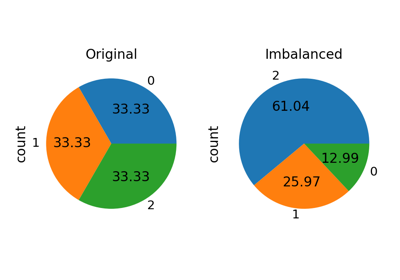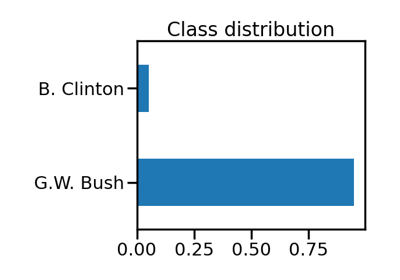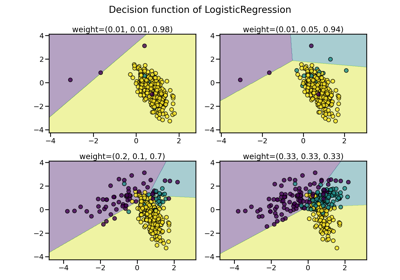# imblearn.over_sampling.RandomOverSampler¶

class imblearn.over_sampling.RandomOverSampler(*, sampling_strategy='auto', random_state=None)[source]

Class to perform random over-sampling.

Object to over-sample the minority class(es) by picking samples at random with replacement.

Read more in the User Guide.

Parameters
sampling_strategyfloat, str, dict or callable, default=’auto’

Sampling information to resample the data set.

• When float, it corresponds to the desired ratio of the number of samples in the minority class over the number of samples in the majority class after resampling. Therefore, the ratio is expressed aswhereis the number of samples in the minority class after resampling andis the number of samples in the majority class.

Warning

float is only available for binary classification. An error is raised for multi-class classification.

• When str, specify the class targeted by the resampling. The number of samples in the different classes will be equalized. Possible choices are:

'minority': resample only the minority class;

'not minority': resample all classes but the minority class;

'not majority': resample all classes but the majority class;

'all': resample all classes;

'auto': equivalent to 'not majority'.

• When dict, the keys correspond to the targeted classes. The values correspond to the desired number of samples for each targeted class.

• When callable, function taking y and returns a dict. The keys correspond to the targeted classes. The values correspond to the desired number of samples for each class.

random_stateint, RandomState instance, default=None

Control the randomization of the algorithm.

• If int, random_state is the seed used by the random number generator;

• If RandomState instance, random_state is the random number generator;

• If None, the random number generator is the RandomState instance used by np.random.

SMOTE

Oversample by generating synthetic samples.

Notes

Supports multi-class resampling by sampling each class independently. Supports heterogeneous data as object array containing string and numeric data.

Examples

>>> from collections import Counter
>>> from sklearn.datasets import make_classification
>>> from imblearn.over_sampling import RandomOverSampler
>>> X, y = make_classification(n_classes=2, class_sep=2,
... weights=[0.1, 0.9], n_informative=3, n_redundant=1, flip_y=0,
... n_features=20, n_clusters_per_class=1, n_samples=1000, random_state=10)
>>> print('Original dataset shape %s' % Counter(y))
Original dataset shape Counter({1: 900, 0: 100})
>>> ros = RandomOverSampler(random_state=42)
>>> X_res, y_res = ros.fit_resample(X, y)
>>> print('Resampled dataset shape %s' % Counter(y_res))
Resampled dataset shape Counter({0: 900, 1: 900})

Attributes
sample_indices_ndarray of shape (n_new_samples)

Indices of the samples selected.

New in version 0.4.

__init__(self, *, sampling_strategy='auto', random_state=None)[source]

Initialize self. See help(type(self)) for accurate signature.

## Examples using imblearn.over_sampling.RandomOverSampler¶